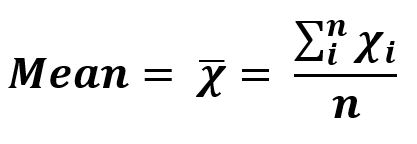# sciPy stats.tmean() | Python

`scipy.stats.tmean(array, limits=None, inclusive=(True, True))` calculates the trimmed mean of the array elements along the specified axis of the array.

It’s formula –Parameters :
array: Input array or object having the elements to calculate the trimmed mean.
axis: Axis along which the trimmed mean is to be computed. By default axis = 0.
limits: Lower and upper bound of the array to consider, values less than the lower limit or greater than the upper limit will be ignored. If limits is None [default], then all values are used.

Returns : Trimmed mean of the array elements based on the set parameters.

Code #1:

 `# Trimmed Mean  ` `  `  `from` `scipy ``import` `stats ` `import` `numpy as np  ` `  `  `# array elements ranging from 0 to 19 ` `x ``=` `np.arange(``20``) ` `   `  `print``(``"Trimmed Mean :"``, stats.tmean(x))  ` `  `  `  `  `print``(``"\nTrimmed Mean by setting limit : "``,  ` `      ``stats.tmean(x, (``2``, ``10``))) `

Output:

```Trimmed Mean : 9.5

Trimmed Mean by setting limit :  6.0
```

Code #2: With multi-dimensional data, axis() working

 `# Trimmed Mean  ` `  `  `from` `scipy ``import` `stats ` `import` `numpy as np  ` ` `  `arr1 ``=` `[[``1``, ``3``, ``27``],  ` `        ``[``5``, ``3``, ``18``],  ` `        ``[``17``, ``16``, ``333``],  ` `        ``[``3``, ``6``, ``82``]]  ` `  `  ` `  `# using axis = 0 ` `print``(``"\nTrimmed Mean is with default axis = 0 : \n"``,  ` `      ``stats.tmean(arr1, axis ``=` `1``)) `

Output:

```Trimmed Mean is with default axis = 0 :
42.8333333333
```

Whether you're preparing for your first job interview or aiming to upskill in this ever-evolving tech landscape, GeeksforGeeks Courses are your key to success. We provide top-quality content at affordable prices, all geared towards accelerating your growth in a time-bound manner. Join the millions we've already empowered, and we're here to do the same for you. Don't miss out - check it out now!

Previous
Next